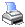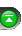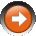﻿ True Strength Index (TSI)
 NinjaScript > Language Reference > Indicator Methods > True Strength Index (TSI)Description

The True Strength Index (TSI) is a momentum-based indicator, developed by William Blau. Designed to determine both trend and overbought/oversold conditions, the TSI is applicable to intraday time frames as well as long term trading.

Syntax

 TSI(int fast, int slow) TSI(IDataSeries input, int fast, int slow)   Returns default value TSI(int fast, int slow)[int barsAgo] TSI(IDataSeries input, int fast, int slow)[int barsAgo]

Return Value

double; Accessing this method via an index value [int barsAgo] returns the indicator value of the referenced bar.

Parameters

 fast The number of bars to include in the calculation input Indicator source data (?) slow The number of bars to include in the calculation

Examples

 // Prints the current value of a 20 period TSI using default price type double value = TSI(20); Print("The current TSI value is " + value.ToString());   // Prints the current value of a 20 period TSI using high price type double value = TSI(High, 20); Print("The current TSI value is " + value.ToString());

Source Code

You can view this indicator method source code by selecting the menu Tools > Edit NinjaScript > Indicator within the NinjaTrader Control Center window.